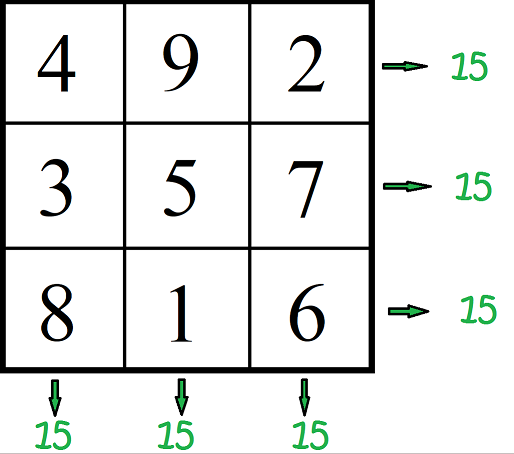# Definition of Magic SquareA magic square is a square divided into smaller squares, each of which must be filled with a whole number so that the sum of the numbers in each row, column and main diagonal is equal to the same amount.

In the example in the picture, all of the rows, columns, and main diagonals add up to the number $15$. In fact, any $3 \times 3$ magic square, filled with the numbers $\{1,2,3,4,5,6,7,8,9\}$ will have its rows, columns and main diagonals sum to $15$.

You can make magic squares of any size: $2\times 2$, $3 \times 3$, $4 \times 4$, $5 \times 5$ and so on.

### Description

The aim of this dictionary is to provide definitions to common mathematical terms. Students learn a new math skill every week at school, sometimes just before they start a new skill, if they want to look at what a specific term means, this is where this dictionary will become handy and a go-to guide for a student.

### Audience

Year 1 to Year 12 students

### Learning Objectives

Learn common math terms starting with letter M

Author: Subject Coach
You must be logged in as Student to ask a Question.